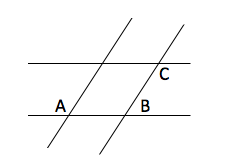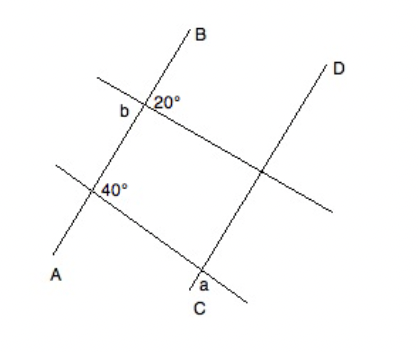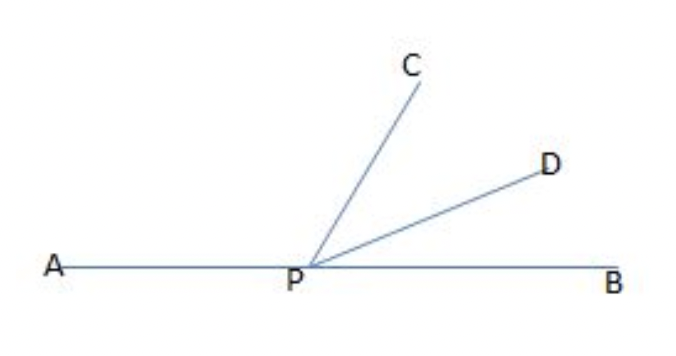# ACT Mathematics: Geometry Quiz

This is an MCQ-based quiz on the topic of ACT Mathematics: Geometry Quiz.

This set of MCQs helps you brush up on the important mathematics topic and prepare you to dive into skill practice and expand your knowledge to tackle the question and answer it carefully.

.

Start Quiz

If ∠A measures (40−10x)∘, which of the following is equivalent to the measure of the supplement of ∠A ?

10x + 50

100x

140 - 10x

10x + 140

Lines AC and BD are parallel.  ∠HFC=10∘, ∠DGI=50∘, △EFG is a right triangle, and  EG has a length of 10.  What is the length of  EF?20

5

15

12

Two pairs of parallel lines intersect:If A = 135o, what is 2*|B-C| = ?

180

140

150

170

A student creates a challenge for his friend.  He first draws a square, the adds the line for each of the 2 diagonals.  Finally, he asks his friend to draw the circle that has the most intersections possible.

10

12

4

6

In the diagram, AB || CD. What is the value of a+b?160

80

60

140

One-half of the measure of the supplement of angle ABC is equal to the twice the measure of angle ABC. What is the measure, in degrees, of the complement of angle ABC?

72

45

54

18

In the figure above, APB forms a straight line. If the measure of angle APC is eighty-one degrees larger than the measure of angle DPB, and the measures of angles CPD and DPB are equal, then what is the measure, in degrees, of angle CPB?50

33

114

66

Two angles are supplementary and have a ratio of 1:4.  What is the size of the smaller angle?

45

18

144

36

Simplify the following fraction:

24/256

6/64

3/32

8/32

12/128

Solve for x.

16/(x+7)=2/6

28

41

24

12

Quiz/Test Summary
Title: ACT Mathematics: Geometry Quiz
Questions: 10
Contributed by: